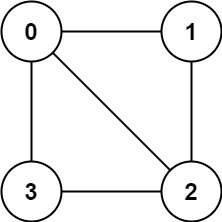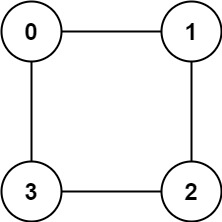# Is Graph Bipartite?

published on 4/29/2022

There is an undirected graph with n nodes, where each node is numbered between 0 and n - 1. You are given a 2D array graph, where graph[u] is an array of nodes that node u is adjacent to. More formally, for each v in graph[u], there is an undirected edge between node u and node v. The graph has the following properties:

There are no self-edges (graph[u] does not contain u). There are no parallel edges (graph[u] does not contain duplicate values). If v is in graph[u], then u is in graph[v] (the graph is undirected). The graph may not be connected, meaning there may be two nodes u and v such that there is no path between them. A graph is bipartite if the nodes can be partitioned into two independent sets A and B such that every edge in the graph connects a node in set A and a node in set B.

Return true if and only if it is bipartite.

#### Example 1:```Input: graph = [[1,2,3],[0,2],[0,1,3],[0,2]]Output: falseExplanation: There is no way to partition the nodes into two independent sets such that every edge connects a node in one and a node in the other.
``````Input: graph = [[1,3],[0,2],[1,3],[0,2]]Output: trueExplanation: We can partition the nodes into two sets: {0, 2} and {1, 3}.
```

### Constraints:

• graph.length == n
• 1 <= n <= 100
• 0 <= graph[u].length < n
• 0 <= graph[u][i] <= n - 1
• graph[u] does not contain u.
• All the values of graph[u] are unique.
• If graph[u] contains v, then graph[v] contains u.

### Intuition:

• If a graph has an odd length cycle, then it's not a bipartite graph.
• If it doesn't have an odd length cycle, then it's a bipartite graph.

### Solution in DFS:

#### Solution explanation:

• Initialize the color array with -1
• We can use a color array to mark the nodes, wether colored or not.
• We can use DFS to check if the graph is bipartite.
• If the node is not colored, then we color its with 1.
• And run DFS on its neighbors.
• If the current node is not colored, then assign it with 1-color[node]
• If the node is colored,and its equal to the previous node color then it's not bipartite.
```class Solution:    def isBipartite(self, graph: List[List[int]]) -> bool:        n = len(graph)        color = [-1 for i in range(n)]        def dfs(node):            if color[node] == -1:                color[node] = 1            for curNode in graph[node]:                if color[curNode] == -1:                    color[curNode] = 1-color[node]                    if(not dfs(curNode)):                        return False                elif color[curNode] == color[node]:                    return False            return True        for i in range(n):            if color[i] == -1:                if(not dfs(i)):                    return False        return True
```

#### Space complexity: O(N+E)+O(N)+O(N)

Created with ❤️ by Avinash Sharma